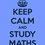# RMO 2014 Delhi Region Q.2

Let $$a_1,a_2,\ldots ,a_{2n}$$ be an arithematic progression of positive real numbers with common difference $$d$$ . Let

$\large\left\{\begin{array}{l}\displaystyle\sum^n_{i=1} a^2_{2i-1}=x\\\ \displaystyle\sum^n_{i=1} a^2_{2i}=y\\\ a_n+a_{n+1}=z\end{array}\right.$

Express $d$ in terms of $x, y, z, n$.

• You can find rest of the problems here

• You can find the solutions hereNote by Aneesh Kundu
6 years, 7 months ago

This discussion board is a place to discuss our Daily Challenges and the math and science related to those challenges. Explanations are more than just a solution — they should explain the steps and thinking strategies that you used to obtain the solution. Comments should further the discussion of math and science.

When posting on Brilliant:

• Use the emojis to react to an explanation, whether you're congratulating a job well done , or just really confused .
• Ask specific questions about the challenge or the steps in somebody's explanation. Well-posed questions can add a lot to the discussion, but posting "I don't understand!" doesn't help anyone.
• Try to contribute something new to the discussion, whether it is an extension, generalization or other idea related to the challenge.

MarkdownAppears as
*italics* or _italics_ italics
**bold** or __bold__ bold
- bulleted- list
• bulleted
• list
1. numbered2. list
1. numbered
2. list
Note: you must add a full line of space before and after lists for them to show up correctly
paragraph 1paragraph 2

paragraph 1

paragraph 2

[example link](https://brilliant.org)example link
> This is a quote
This is a quote
    # I indented these lines
# 4 spaces, and now they show
# up as a code block.

print "hello world"
# I indented these lines
# 4 spaces, and now they show
# up as a code block.

print "hello world"
MathAppears as
Remember to wrap math in $$ ... $$ or $ ... $ to ensure proper formatting.
2 \times 3 $2 \times 3$
2^{34} $2^{34}$
a_{i-1} $a_{i-1}$
\frac{2}{3} $\frac{2}{3}$
\sqrt{2} $\sqrt{2}$
\sum_{i=1}^3 $\sum_{i=1}^3$
\sin \theta $\sin \theta$
\boxed{123} $\boxed{123}$

Sort by:

$\displaystyle d = \frac{y-x}{nz}$

- 6 years, 7 months ago

got the same answer yesterday in RMO

- 6 years, 7 months ago

Hi Sudeep, Any tips for studying coordinate geometry for JEE?

- 6 years, 7 months ago

Got the same question in Tamil Nadu's RMO. My approach was as follows:

$y-x=(a^{ 2 }_{ 2n }-a^{ 2 }_{ 1 })+(a^{ 2 }_{ 2n-2 }-a^{ 2 }_{ 3 })+...+(a^{ 2 }_{ 2 }-a^{ 2 }_{ 2n-1 })$

It is a property of an AP that the sum of equidistant terms from the middle term(s) is a constant. Note that $a_n$ and $a_{n+1}$ are the middle terms of this AP. Hence, their sum $z$ is constant for all equidistant terms.

$y-x=(a_{ 2n }+a_{ 1 })(a_{ 2n }-a_{ 1 })+(a_{ 2n-2 }+a_{ 3 })(a_{ 2n-2 }-a_{ 3 })+...+(a_{ 2 }+a_{ 2n-1 })(a_{ 2 }-a_{ 2n-1 })$

$y-x=z(a_{ 2n }-a_{ 1 }) + z(a_{ 2n-2 }-a_{ 3 }) + ... + z(a_{ 2 }-a_{ 2n-1 })$

$y-x=z(a_{ 2n }-a_{ 1 } + a_{ 2n-2 }-a_{ 3 } +a_{ 2 }-a_{ 2n-1 })$

$y-x=z ((a_{2n}-a_{2n-1}) + (a_{2n-2} - a_{2n-3}) + ... + (a_2-a_1))$

The above terms are the difference of two consecutive terms, $d$, and there are $n$ such terms:

$y-x=znd$

$d=\dfrac{y-x}{zn}$

- 6 years, 7 months ago

its same as in RMO Karnataka Region!

I got a equation with x,y,z,n,d and d^2.. Couldn't go further!

- 6 years, 7 months ago

I think i got it correct.

- 6 years, 7 months ago

Well,what is it? How many did you solve in total?

- 6 years, 7 months ago

4

- 6 years, 7 months ago

I don't know whether my answer is right or not

- 6 years, 7 months ago

Did u try using quadratic formula after that?

- 6 years, 7 months ago

No! I didn't. May be the equation was wrong, Sudeep Salgia's solution looks right!

- 6 years, 7 months ago

See my comment in the discussion.

- 6 years, 7 months ago

In an AP if a1+a5+a10+a15+a25=300, find sum up to 24 terms?

- 6 years, 6 months ago

(Y- x)/z

- 6 years, 7 months ago

Why?

- 6 years, 7 months ago